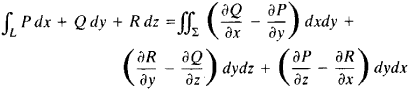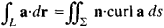# Stokes Theorem

## Stokes’ Theorem

a theorem giving a formula for the conversion of a line integral around a closed curve L into the surface integral over the surface Σ bounded by L. The theorem states thatThe direction in which L is traversed in taking the line integral must be coordinated with the orientation of Σ.

In vector form, Stokes’ theorem readswhere a = Pi + Qj + Rk, dr is a linear element of L, ds is an element of area of Σ, and n is the unit normal to Σ.

The physical significance of Stokes’ theorem is that the circulation of a vector field around L is equal to the flux of vorticity of the field through Σ. Stokes’ theorem was set forth by G. G. Stokes in 1854.

Site: Follow: Share:
Open / Close